Math Concepts
Measuring Units
18.8k views

## Measuring Units

Measurement is to find a number that shows the amount of something.

 1 What is a measurement? 2 What is a measuring unit? 3 Lengths of given objects 4 Weights of given objects 5 Money 6 Time 7 Conclusion 8 Frequently Asked Questions (FAQs) 9 External References

## What is a measuring unit?

A measuring unit is a standard quantity used to express a physical quantity. Let us learn about the physical quantities and some of the standard units used to measure them.

The Physical quantities are further classified into 4 types:
1.     Lengths of given objects
2.     Weights of given objects
3.     Money
4.     Time

### 1. Lengths of given objects

Length describes how long a thing is from one end to the other. Length is used to identify the size of the object or a distance from one point to another.

 Metric Unit US Standard Unit (English Unit or Customary Unit) Millimetre (mm): Used to measure very short lengths or thicknesses. Example: length of a pen tip. Inch (in): Used to measure the length of small objects. Example: Length of a rod. Centimetre (cm): Used to measure small lengths. Example: Length of a pen. Foot (ft): Used to measure short distances and heights. Example: Heights of buildings. Meter (m): Used to measure big lengths. Example: Length of a classroom. Yard (yd): It is bigger than a foot. Example: Length of a football field. Kilometre (km): Used to measure very long lengths or distance. Example: Distance between two places. Mile (mi): Used to measure long distances. Example: Distance between two places.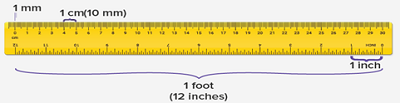The object can be differentiated or compared by its length.
Here’s an image showing 3cm length.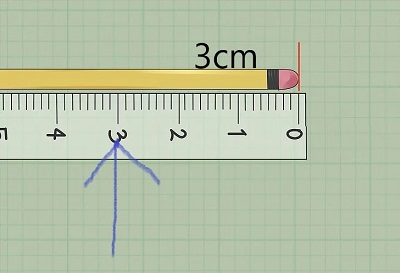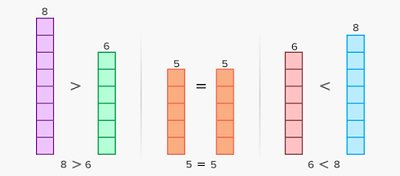The above example is an illustration of how the object is compared by its length.
Here are few conversions of length:
1.     1 km = 1000m
2.     1m = 100 cm.     1cm = 10mm

### 2. Weights of given objects

Metric Unit US Standard Unit (English Unit or Customary Unit) Millimetre (mm): Used to measure very light things. Example: Medicines Ounce (oz): Used to measure small quantities. Example: Bread. Gram (g):  Used to measure small things. Example: Potato Pound (lb): Used to measure body weight, etc. Example: Bread Kilogram (kg):  Used to measure heavy things. Example: Bodyweight. Ton: Used to measure much heavier things. Example: Trucks and heavy load

### Measuring Units-PDF

Cuemath’s learning platform has carefully designed simulations to help the student visualize concepts. This blog will help us understand about the measuring units. Here is a downloadable PDF to explore more.

Here are few conversions of weight:
1.     1 kg = 1000 grams.
2.     1 gram = 1000 milligrams.
3.     1 gram = (1/1000) kg.
4.     1 milligram = (1/1000) gram.
The object can be compared with its weight.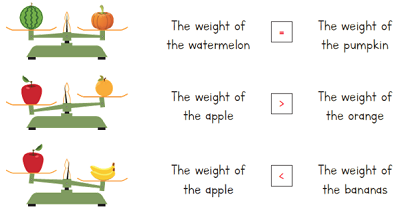The above example is an illustration of how an object is compared by its weight.
Note: The heavier object weighs down.

3. Money

Money can be defined as anything that people use to buy goods and services. Money is a part of everyone’s life.
Since the money/currency differs for each and every country because each and every country use their own currencies. Each country's currency value differs from other countries on the basis of their economy.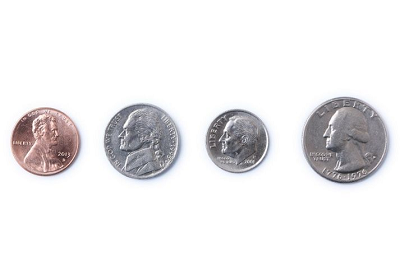Country US Standard Unit (English Unit or Customary Unit) India Indian Rupees. United States United States Dollars. United Kingdom. Pound. Japan Japanese Yen. Australia Australian dollar. Russia Russian Rouble.

Here are few currency conversions:
One U.S Dollar = 74.74 rupee.
One Pound = 96.97 rupee.
One Rupee = 1.40 Japanese yen.
According to Indian currency:

• ₹1 = 100 paisa

• 1 paisa = (1/100) rupee

4. Time

The ongoing sequence of events is time.
The basic unit of time is the second.
There are also minutes, hours, days, weeks, months and years.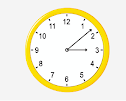Metric Units and US Standard Unit are the same for the time

Second (s)

Minute (min)

Hour (hr)

Day

Week

Month

Yea

Whereas,

• 1 year = 12 months

• 1 month = 4 weeks approx.

• 1 week = 7days.

• 1 day = 24 hours.

• 1 hour = 60 minutes

• 1 minute = 60 seconds.

Measuring units is a basic concept which we come across in our daily life. Teaching kids about the measuring unit such as length, weight, money and time give them a good idea and will let the kid explore more.

It gives a proper image to the kids in comparing the objects based on the units because kids must understand the importance of measurement and be familiar with their use in everyday life.  It is an essential life skill.

External References

Cuemath, a student-friendly mathematics and coding platform, conducts regular Online Classes for academics and skill-development, and their Mental Math App, on both iOS and Android, is a one-stop solution for kids to develop multiple skills. Understand the Cuemath fee structure and sign up for a free trial.

## What are the 7 basic units of measurement?

• Length - meter (m)
• Time - second (s)
• Amount of substance - mole (mole)
• Electric current - ampere (A)
• Temperature - kelvin (K)
• Luminous intensity - candela (cd)
• Mass - kilogram (kg)

## Is SI an unit?

The International System of Units (SI, abbreviated from the French Système international (d'unités)) is the modern form of the metric system. It is the only system of measurement with an official status in nearly every country in the world.

Related Articles